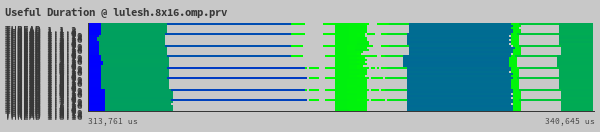# Sequential communications in hybrid programming

A frequent practice in hybrid programming is to only parallelize with OpenMP the main computational regions. The communication phases are left as in the original MPI program and thus execute in order in the main thread while other threads are idling. This may limit the scalability of hybrid programs and often results in the hybrid code being slower than an equivalent pure MPI code using the same total number of cores.

In many cases several communication follow a computation phase. The data produced in it is packed into buffers before sending (or unpacked after receiving). If the domain compunted in the process has several neighbours, several such communications will be done, typically in sequence. Depending on the size od the messages, the packing/unpacing may take some significant cost (several milliseconds). This typical practice executes serially those operations within the critical path.

Many variants of source code structure for this type of issue can be seen in real codes, but a possible mockup source code structure reflecting this is shown in the following code listing:

#pragma omp parallel for
for (i=0; i<SIZE; i++) compute(data);

for (n=0; n<n_neighs; n++) {
MPI_irecv(&r_buff[n], n, ...)

pack(s_buff[n], data, n ); // packing send buffer for neighbor 'n'
MPI_Isend(&s_buff[n], n, ...);
}
MPI_Waitall();

for (n=0; n<n_neighs; n++) {
unpack(r_buff[n], data, n); // unpacking receive buffer for neighbor 'n'
}


An example of this pattern occurs in the LULESH code. The following timeline shows how internal rank threads are underused when executing the communication phases.The typical packing operation to prepare data for MPI send calls or unpacking the received data are often embedded inmediately preceding/following the corresponding MPI call in tight sequences of potentially many communications. This introduces the cost of such operations in the critical path and potentially delays the actual data transfers. In many cases these pack/unpack operations are independent between themselves and from other communication calls in the sequence except the one directly targetted by it.

Recommended best-practice(s):